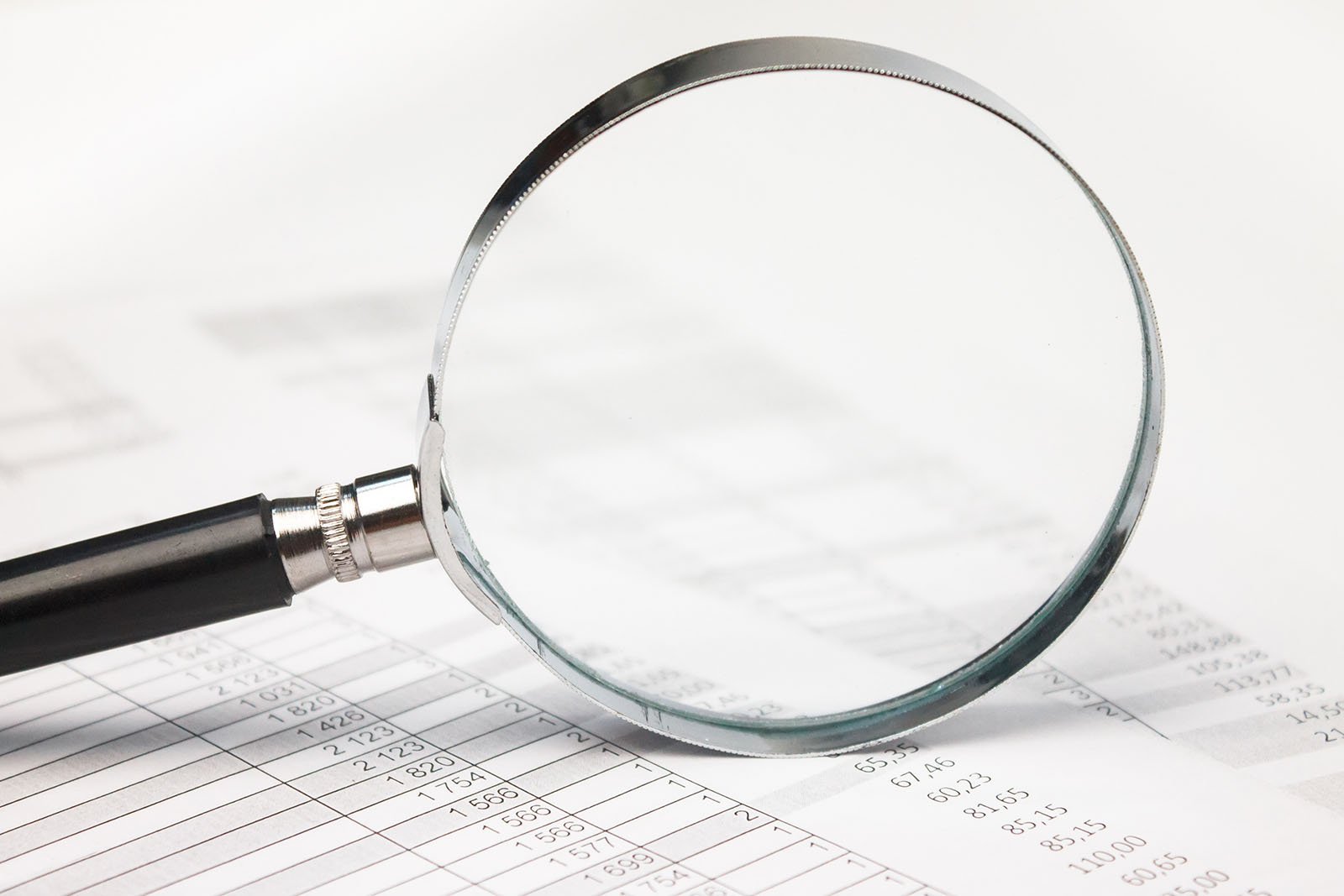Malin Edvardsson Dec 22, ’22 < 8 min

# QCM parameters – this is what they mean

## Surface Science Blog

Reading and understanding a QCM specification can be tricky. In this post we explain what different parameters mean, and which ones that are relevant to keep an eye on.

## Which are the important parameters?

The key parameter measured by a QCM instrument is the resonance frequency change of the oscillator. Extended QCM setups will in addition to the frequency change, also measure one or more parameters related to the energy losses in the system. This seems straightforward, but the parameters included in an instrument specification can both vary and be described with different terminology.

So, which of all the parameters listed in a specification are relevant and will have an impact on the end measurement? And how can these be compared between different instrument suppliers? Below we have collected some of the parameters that are often mentioned in QCM specifications and explain what they mean.

UNIT

### RESOLUTION

Time

The time resolution, or sampling rate, describes how many data points that are collected per second. The higher the sampling rate, the faster kinetic processes can be resolved in an experiment.

Hz

Frequency

The frequency resolution is basically the number of decimals the instrument can display. A high reso­lution does not necessarily mean that small frequency changes can be detected; the extent to which the displayed numbers are significant depends on the noise level. As long as the frequency resolution is better than the noise level, which means that it is possible to resolve the changes taking place, it is not relevant for a real measurement situation. Example: If, for example, only two decimals are significant (above the noise level), it does not matter if the resolution is 10 decimals or 15.

Hz

Dissipation

Same as above

unitless

Mass

The mass resolution correlates with the frequency resolution (see above) by a multiplication of the constant sensitivity factor (for a 5 MHz sensor, this is 17.7 ng/(cm2∙Hz)). As long as the resolution is better than the noise level, i.e., you are able to resolve the changes taking place, this parameter is not relevant for a real measurement situation.

ng/cm²

Temperature

The temperature resolution describes how exact the actual temperature can be measured. As long as the temperature resolution is better than the stability level, i.e., that it is possible to measure the temperature changes taking place, it has no relevance for a real measurement situation.

˚C

### SENSITIVITY

Frequency

The useful frequency sensitivity is the smallest significant change that can be resolved in the frequency signal. The sensitivity almost always depends more on the noise level than on how many decimals that can be displayed. I.e. it is the noise level, which in the end will determine how many of the displayed decimals of the frequency parameter that are significant. Example: the instrument has a specified signal resolution of 0.001 Hz, and a noise level of 0.05 Hz. Assuming you accept a signal-to-noise ratio of 2, the useful frequency sensitivity will be 0.1 Hz

Hz

Mass (theoretical)

The theoretical mass sensitivity depends on the crystal fundamental mode . Example: For a 5 MHz crystal it is 17.7 ng/(cm2∙Hz) The extent to which the theoretical mass sensitivity is useful is determined by the useful frequency sensitivity, i.e. the smallest significant change in frequency signal that can be resolved.

ng/(cm²∙Hz)

Mass (useful)

The useful mass sensitivity, i.e. the sensitivity which can be achieved in an experimental situation, equals the theoretical mass sensitivity multiplied by the useful frequency sensitivity. Example: With a 5 MHz crystal, the theoretical sensitivity is 17.7 ng/(cm2∙Hz). With a useful frequency sensitivity of 0.1 Hz, the useful mass sensitivity will be 1.8 ng/cm2.

ng/cm²

Dissipation

The useful dissipation sensitivity is the smallest significant change that can be resolved in the Dissipation signal. The useful sensitivity almost always depends more on the noise level than on how many decimals that can be displayed.

unitless

### NOISE

Frequency

The noise describes uncontrolled, short-term variations, of the signal. The noise will determine how much of the measured signal that can be utilized and determine how many of the displayed decimals that are significant.

Hz

Dissipation

Same as above

unitless

Temperature

Same as above

˚C

### LONG-TERM STABILITY

Frequency

Frequency stability, or drift, describes long-term variations of the signal. A stable signal is important, espe­cially for longer measurements. High drift will make the results ambiguous, and it will be unclear whether the measured frequency response reflects mass changes or if it is due to undefined instrument variations.

Hz/h

Dissipation

Same as above

1/h

Temperature

The temperature stability describes the temperature drift per time unit. Since the temperature affects the QCM signals, a good temperature control and stability is essential for reliable measurement results.

˚C/h

## Concluding remarks

Understanding and comparing different QCM specifications can be a challenge. Not only are the parameters used often described in different ways which makes it difficult to do a direct comparison, but it can also be tricky to decipher how the parameters mentioned relate to for example the data quality. In this post, we have listed the key parameters, explained what they mean, and how they relate to the quality of the data produced.Guide## Explore the blog

You have only scratched the surface.